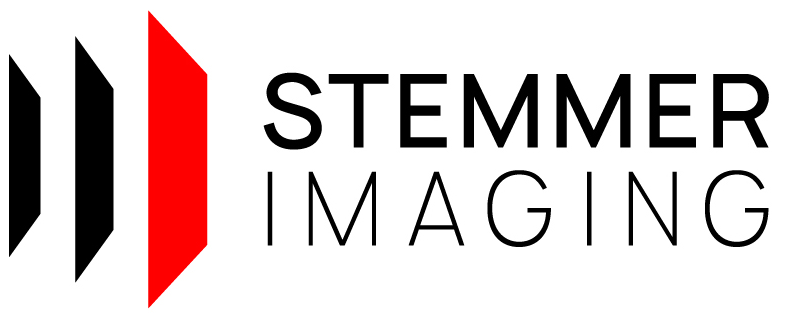CVB.Net 14.0
PointCloudExtensions Class Reference

Extension methods for PointClouds. More...

## Public Types

enum  CropRange { CropWithinRange = 0 , CropOutsideRange = 1 }
Indicates cropping range. More...

enum  CropDirection { Below = 0 , Above = 1 }
Indicates cropping direction. More...

## Static Public Member Functions

static Cuboid CalculateBoundingBox (this PointCloud pc)
Calculates the minimum and maximum extent of the point cloud. More...

static Point3Dd CalculateCenterOfGravity (this PointCloud pc)
Calculates the center of gravity of the point cloud. More...

static Matrix3D CalculateCovarianceMatrix (this PointCloud pc)
Calculates the covariance matrix of the point cloud. More...

static Geometry3D.Plane FitPlane (this PointCloud pc)
Fits a plane in the points of the PointCloud pc . More...

static Geometry3D.Plane FitPlane (this PointCloud pc, Cuboid aoi)
Fits a plane in the points of the PointCloud pc restricted by the given aoi . More...

static PointCloud Crop (this PointCloud pc, Cuboid clipBox)
Creates a new point cloud which is a copy of this point cloud, which only consists of the points inside the given clipBox . More...

static PointCloud FrustumCrop (this PointCloud pc, Cuboid clipBox, Angle theta, Angle phi)
Creates a new point cloud which only cosists of the points inside the clipBox , where the orientation of the two boundary planes Z=Zmin and Z=Zmax can be modified by angles theta and phi . More...

static PointCloud PlaneCrop (this PointCloud pc, Geometry3D.Plane plane, ValueRange< double > range, CropRange cropRange)
Creates a new point cloud where points within or outside a range parallel to given plane are cropped. More...

static PointCloud PlaneCrop (this PointCloud pc, Geometry3D.Plane plane, double threshold, CropDirection cropBelowAbove=CropDirection.Below)
Creates a new point cloud which only consists of the points below or above given plane . More...

static PointCloud DownsampleByFactor (this PointCloud pc, int factor)
Creates a new point cloud which is a copy of this point cloud, with every nth point removed (n being defined by the given factor ). More...

static PointCloud DownsampleRandomly (this PointCloud pc, int amount)
Creates a new point cloud which is a copy of this point cloud, with n random points removed (n being defined by the given amount ). More...

static PointCloud Scale (this PointCloud pc, Factors3D factors)
Creates a new point cloud which is a copy of this point cloud, with all points scaled by the given factors . More...

static Image ToRangeMap (this PointCloud pc, ValueRange< double > xRange, ValueRange< double > yRange, Size2D targetSize, double backgroundValue)
Creates a new range map image via linear project in negative z direction. More...

static Image ToRangeMap (this PointCloud pc, ValueRange< double > xRange, ValueRange< double > yRange, int targetWidth, int targetHeight, double backgroundValue)
Creates a new range map image via linear project in negative z direction. More...

static void Save (this PointCloud pc, string fileName)
Saves this point cloud to the given fileName . More...

static byte[] ToMemory (this PointCloud pc, PointCloudFlags flags, FileFormat fileFormat)
Saves this point cloud to memory. More...

static void ToMemory (this PointCloud pc, IntPtr buffer, long bufferSize, PointCloudFlags flags, FileFormat fileFormat)
Saves this point cloud to the given buffer . More...

## Detailed Description

Extension methods for PointClouds.

## ◆ CropDirection

 enum CropDirection

Indicates cropping direction.

Enumerator
Below

Crop below given plane.

Above

Crop above given plane.

## ◆ CropRange

 enum CropRange

Indicates cropping range.

Enumerator
CropWithinRange

Crop points within given range.

CropOutsideRange

Crop points outside given range.

## ◆ CalculateBoundingBox()

 static Cuboid CalculateBoundingBox ( this PointCloud pc )
static

Calculates the minimum and maximum extent of the point cloud.

Parameters
 pc PointCloud whose bounding box to calculate.
Exceptions
 CvbException When calculating the bounding box failed.
Returns
The bounding box.

## ◆ CalculateCenterOfGravity()

 static Point3Dd CalculateCenterOfGravity ( this PointCloud pc )
static

Calculates the center of gravity of the point cloud.

Parameters
 pc PointCloud whose center of gravity to calculate.
Exceptions
 CvbException When calculating the center of gravity failed.
Returns
Point to the center of gravity.

## ◆ CalculateCovarianceMatrix()

 static Matrix3D CalculateCovarianceMatrix ( this PointCloud pc )
static

Calculates the covariance matrix of the point cloud.

Parameters
 pc PointCloud whose covariance matrix to calculate.
Exceptions
 CvbException When calculating the covariance matrix failed.
Returns
The covariance matrix.

## ◆ Crop()

 static PointCloud Crop ( this PointCloud pc, Cuboid clipBox )
static

Creates a new point cloud which is a copy of this point cloud, which only consists of the points inside the given clipBox .

Parameters
 pc PointCloud to crop. clipBox Cuboid defining the inclusive bounds for the points to be copied.
Returns
Cropped point cloud.
Exceptions
 CvbException When creating the cropped point cloud failed.

## ◆ DownsampleByFactor()

 static PointCloud DownsampleByFactor ( this PointCloud pc, int factor )
static

Creates a new point cloud which is a copy of this point cloud, with every nth point removed (n being defined by the given factor ).

Parameters
 pc PointCloud to downsample. factor Factor by which to remove points.
Returns
Downsampled point cloud.

## ◆ DownsampleRandomly()

 static PointCloud DownsampleRandomly ( this PointCloud pc, int amount )
static

Creates a new point cloud which is a copy of this point cloud, with n random points removed (n being defined by the given amount ).

Parameters
 pc PointCloud to downsample. amount Amount of points to remove.
Returns
Downsampled point cloud.

## ◆ FitPlane() [1/2]

 static Geometry3D.Plane FitPlane ( this PointCloud pc )
static

Fits a plane in the points of the PointCloud pc .

Also fits a plane in points lying on one line, thus make sure that the points really span a plane.

Parameters
 pc Point cloud to extract points from.
Returns
Newly created Plane object fitted in the points from the point cloud.

## ◆ FitPlane() [2/2]

 static Geometry3D.Plane FitPlane ( this PointCloud pc, Cuboid aoi )
static

Fits a plane in the points of the PointCloud pc restricted by the given aoi .

Also fits a plane in points lying on one line, thus make sure that the points really span a plane.

Parameters
 pc Point cloud to extract points from. aoi Bounding cuboid to extract points from.
Returns
Newly created Plane object fitted in the points from the point cloud.

## ◆ FrustumCrop()

 static PointCloud FrustumCrop ( this PointCloud pc, Cuboid clipBox, Angle theta, Angle phi )
static

Creates a new point cloud which only cosists of the points inside the clipBox , where the orientation of the two boundary planes Z=Zmin and Z=Zmax can be modified by angles theta and phi .

For theta , phi = 0,0 the function will give the same result as Crop(PointCloud, Cuboid). Angle theta tilts the two Z-boundary planes towards the (positive) Y-axis. Additionally, phi rotates the tilted Z-boundary planes around the Z-axis (for theta =0, phi will have no effect, because it is then just an inplane-rotation). The two Z-boundary planes will stay parallel, but no longer necessarily orthogonal to the lateral boundary planes. Note, that only the Z-boundaries are tilted and not the whole bounding box, i.e. X- and Y-boundaries will stay the same.

Parameters
 pc PointCloud to frustum crop clipBox ClipBox Cuboid defining the bounds for the points to be copied (min/max are inclusive) theta Theta Tilt angle of the two Z-boundary planes Z=Zmin and Z=Zmax towards the positive Y-axis. phi Phi Rotation angle of the tilted Z-boundary planes around the Z-axis. If Theta = 0, Phi will have no effect.
Returns
Frustum cropped point cloud.

## ◆ PlaneCrop() [1/2]

 static PointCloud PlaneCrop ( this PointCloud pc, Geometry3D.Plane plane, double threshold, CropDirection cropBelowAbove = `CropDirection.Below` )
static

Creates a new point cloud which only consists of the points below or above given plane .

The plane is defined by the hessian normal form: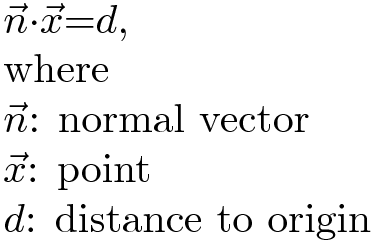Above plane means all points with z values greater than the plane are cropped.It makes no difference in which direction the given normal points. The normal is always converted, so that it points up (positive z value). Note, if Normal.Z equals 0, "below" and "above" is undefined and thus no cropping is done.Instead the input point cloud will be duplicated.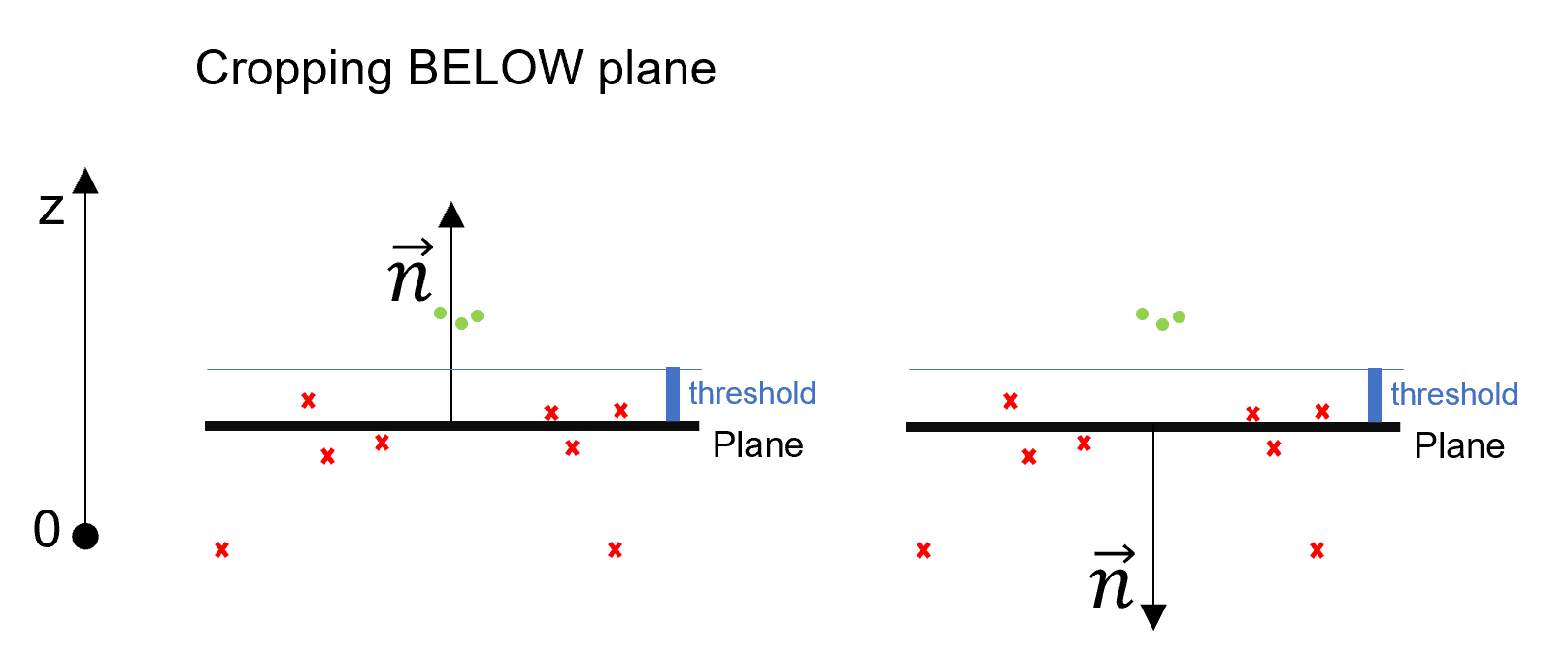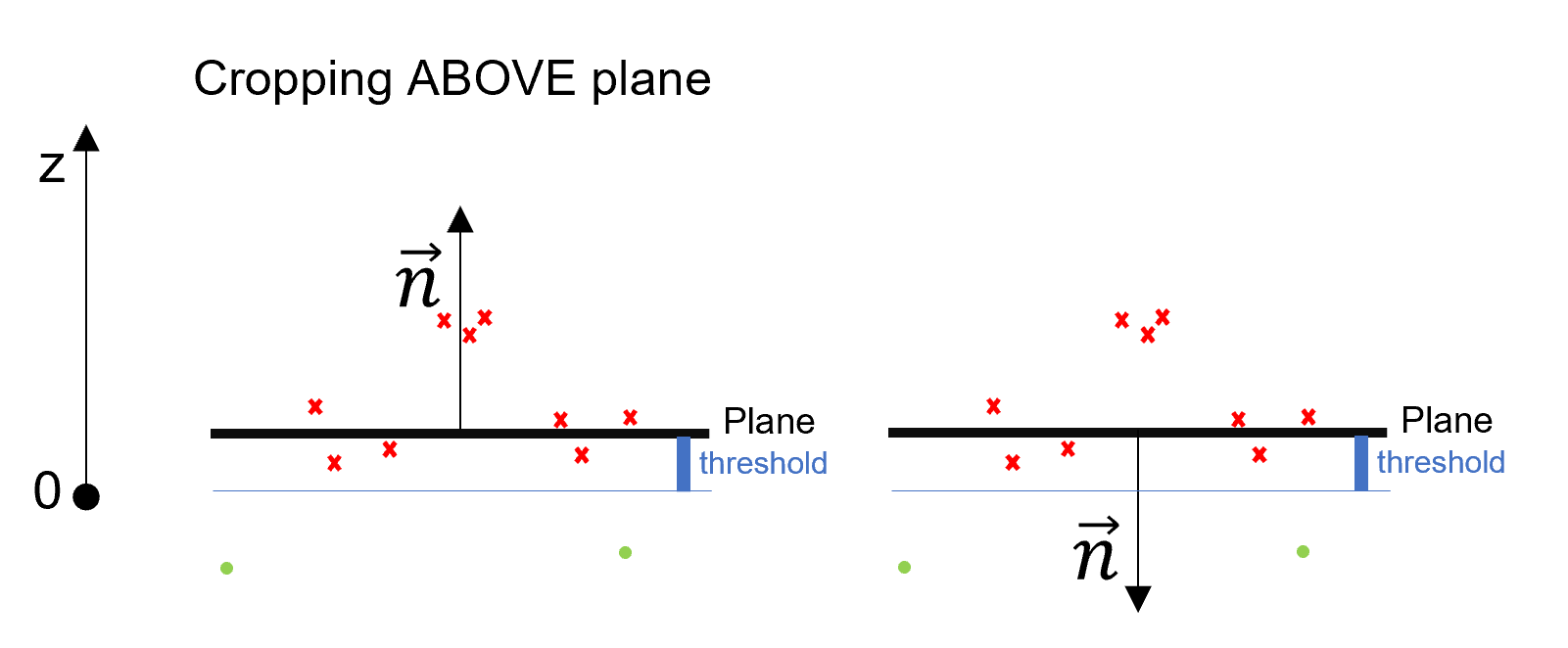If the input point cloud is dense, points beyond the plane are set non-confident.Lines and columns at the borders containing only non-confident points are removed.If the input point cloud is dense and does not contain a confidence plane, a confidence plane will be added
to the cropped point cloud.

Parameters
 pc PointCloud to crop. plane Points beyond this plane will be cropped. threshold Points below plane plus this value and points above plane minus this value are cropped respectively. This threshold should be set, if plane is calculated via FitPlane(). It ensures, that all points beyond this plane plus noise are cropped. cropBelowAbove Indicates if points should either be cropped below or above plane(optional, default value: CropDirection.Below).
Returns
Cropped point cloud.

## ◆ PlaneCrop() [2/2]

 static PointCloud PlaneCrop ( this PointCloud pc, Geometry3D.Plane plane, ValueRange< double > range, CropRange cropRange )
static

Creates a new point cloud where points within or outside a range parallel to given plane are cropped.

The plane is defined by the hessian normal form:Points are cropped within or outside a range parallel to the given plane . The range is defined by range . Note, that range.Max and range.Min are applied along normal direction.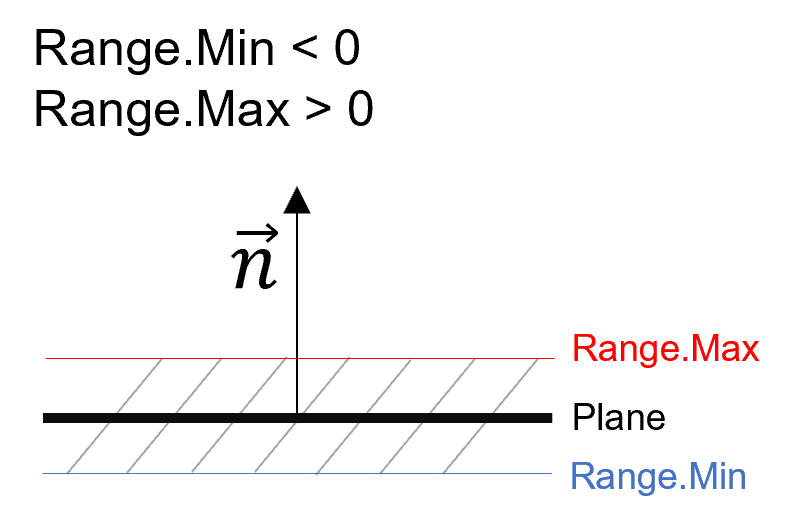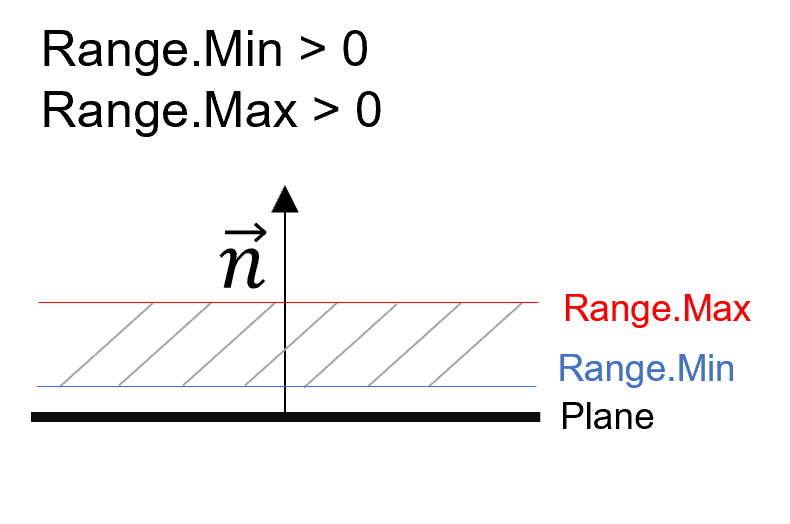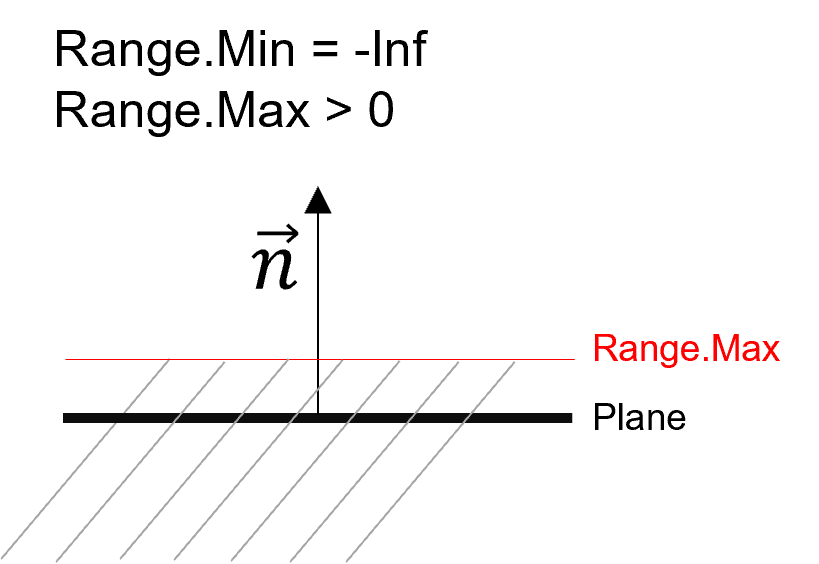In order to define a range around the plane, maximum and minimum range have to be positive and negative respectively.

If the input point cloud is dense, cropped points are set non-confident. Lines and columns at the borders containing only non-confident points are removed. If the input point cloud is dense and does not contain a confidence plane, a confidence plane will be added to the cropped point cloud.

Parameters
 pc PointCloud to crop. plane Points parallel to this plane will be cropped. range Points within or outside this range will be cropped. cropRange Indicates whether points should be cropped within or outside the range.
Returns
Cropped point cloud.

## ◆ Save()

 static void Save ( this PointCloud pc, string fileName )
static

Saves this point cloud to the given fileName .

Parameters
 pc PointCloud to save. fileName Full path to the file to save to.
Exceptions
 CvbException When saving the point cloud failed.

## ◆ Scale()

 static PointCloud Scale ( this PointCloud pc, Factors3D factors )
static

Creates a new point cloud which is a copy of this point cloud, with all points scaled by the given factors .

Parameters
 pc PointCloud to scale. factors Factors to be applied to each point.
Returns
Scaled point cloud.
Exceptions
 CvbException When creating the scaled point cloud failed.

## ◆ ToMemory() [1/2]

 static void ToMemory ( this PointCloud pc, IntPtr buffer, long bufferSize, PointCloudFlags flags, FileFormat fileFormat )
static

Saves this point cloud to the given buffer .

Parameters
 pc Point cloud to save. buffer Buffer to save point cloud to. bufferSize Size of the buffer . flags Flags of the point cloud. fileFormat File format to save.
Exceptions
 CvbException When an error during saving occurs.

## ◆ ToMemory() [2/2]

 static byte[] ToMemory ( this PointCloud pc, PointCloudFlags flags, FileFormat fileFormat )
static

Saves this point cloud to memory.

Parameters
 pc Point cloud to save. flags Flags of the point cloud. fileFormat File format to save.
Returns
Array containing the byte data.
Exceptions
 CvbException When an error during saving occurs.

## ◆ ToRangeMap() [1/2]

 static Image ToRangeMap ( this PointCloud pc, ValueRange< double > xRange, ValueRange< double > yRange, int targetWidth, int targetHeight, double backgroundValue )
static

Creates a new range map image via linear project in negative z direction.

Parameters
 pc PointCloud to create range map from. xRange Input window along the x axis (inclusive). yRange Input window along the y axis (inclusive). targetWidth Target width of the RangeMap in pixels (greater or equal to 2). targetHeight Target height of the RangeMap in pixels (greater or equal to 2). backgroundValue Background value (initialization value for RangeMap).
Returns
The created range map image.
Exceptions
 CvbException When creating the range map failed.

## ◆ ToRangeMap() [2/2]

 static Image ToRangeMap ( this PointCloud pc, ValueRange< double > xRange, ValueRange< double > yRange, Size2D targetSize, double backgroundValue )
static

Creates a new range map image via linear project in negative z direction.

Parameters
 pc PointCloud to create range map from. xRange Input window along the x axis (inclusive). yRange Input window along the y axis (inclusive). targetSize Target size of the RangeMap in pixels (greater or equal to 2). backgroundValue Background value (initialization value for RangeMap).
Returns
The created range map image.
Exceptions
 CvbException When creating the range map failed.